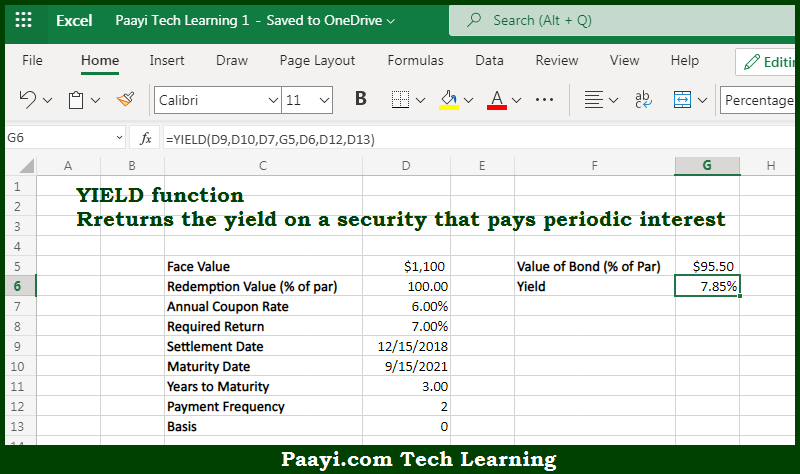# Learn How to Use Microsoft Excel YIELD Function

Written by | 0 Comments | 606 Views

In this article, you will learn how to use the Microsoft Excel YIELD function and its prime function in Microsoft Excel. You will also get to know the Microsoft Excel YIELD function return value and syntax with the help of some examples.

Microsoft Excel YIELD Function

The main purpose of the Microsoft Excel YIELD function is to get the yield for security that pays periodic interest. That implies, with the help of the YIELD function you can able to return the yield on a security that pays periodic interest. So, with the help of the YIELD function, you can able to get the yield for security that pays periodic interest.

Return Value of YIELD Function

The return value will be the yield in the form of a percentage.

Syntax of YIELD Function

=YIELD(sd, md, rate, pr, redemption, frequency, [basis])

Where the arguments:

• sd: This is the settlement date of the security.
• md: This is the maturity date of the security.
• rate: This is the annual coupon rate.
• pr: This is the price of a security per \$100 face value.
• redemption: This is the redemption value per \$100 face value.
• frequency: This is the coupon payments per year, where annual = 1, semiannual = 2, and quarterly = 4.
• basis: This is the day count basis, and the default is 0 (optional).

How to Use Microsoft Excel YIELD Function?So we know that Microsoft Excel YIELD function you can able to get the yield for security that pays periodic interest. That implies, with the help of the YIELD function you can able to return the yield on a security that pays periodic interest. So, with the help of the YIELD function, you can able to get the yield for security that pays periodic interest.